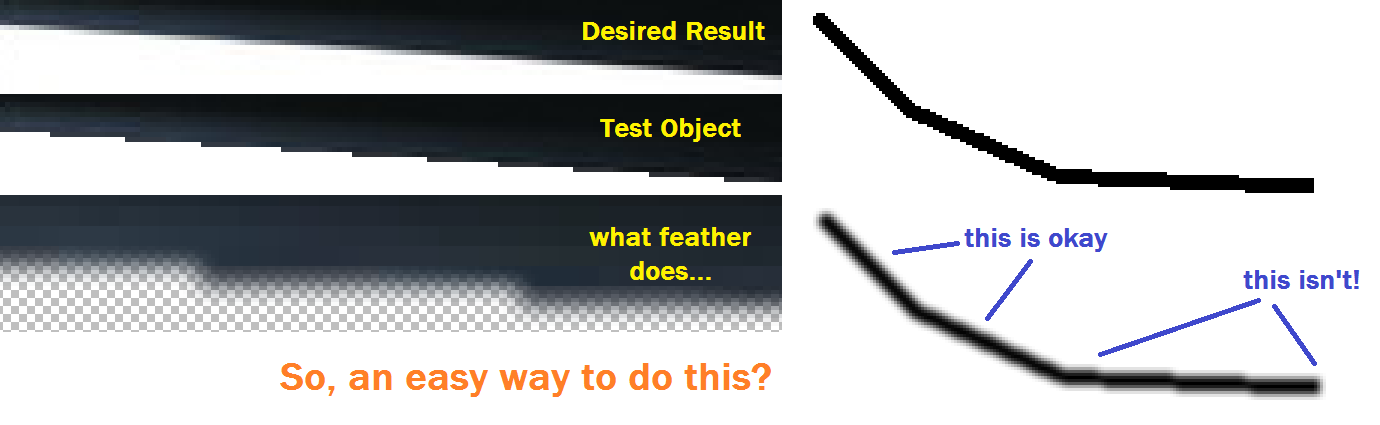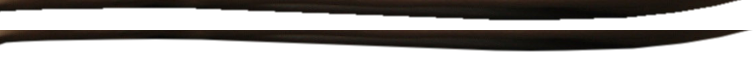# Specific feather option to create transparent edges? (Solved)

## Recommended Posts

Hello, I've been googling around a lot, but couldn't find a way to do what I want.
I work with objects on a transparent background and want to use a feather tool to make the edges soft.
This works fine with pixels close to eachother, but when there are longer lines (closer to horizontal/vertical) this doesn't seem to do the job right.
It doesn't hide the edges, it just stretches and fades them, resulting in a final image where you could still see the way the edges were before (see demo on right side).

I tried some plugins regarding feather, AA etc, but none worked like I wanted.
Is there a tool/plugin that specifically does what I want, or a trick to accomplish it?
See the image below as a demonstration for the issue.Edited by Darty
##### Share on other sites

•2

No, Paint.NET is not spyware...but, installing it is an IQ test. ~BoltBait

Blend modes are like the filling in your sandwich. It's the filling that can change your experience of the sandwich. ~Ego Eram Reputo

##### Share on other sites

Hey @Darty,

Here's what I did:

1) Ran Median () at default values
2) Ran the AA's_Assistant plugin at default values(September 25th, 2023)  Sorry about any broken images in my posts. I am aware of the issue.My Gallery  |  My Plugin Pack

##### Share on other sites

Hey @Darty,

Here's what I did:

1) Ran Median () at default values

2) Ran the AA's_Assistant plugin at default values

Still not really what I'm looking for. It keeps a wavy form, while I want to have it like I demonstrated on top ("Desired Result"). It should somewhat fade over a length of pixels till the end of the 'line', instead of making transparent pixels around it, which keeps the same form eventually.

##### Share on other sites

Here's another way:##### Share on other sites

Here's another way:

Isn't this a way to 'extract' an object from an image within a circle or any form with faded border?

I don't see how this applies to the situation when you already have an object on a transparent background (same layer, deleted image background) and want to fade/feather the object outline.

Feather works for the biggest part like shown before, just with those horizontal/vertical lines it's ugly.

Any more ideas?

##### Share on other sites

You have two options.

@pyjo provided the source code on that page. You can Copy & Paste it into CodeLab.

The Basic Antialias plugin won't solve your issue though.

(September 25th, 2023)  Sorry about any broken images in my posts. I am aware of the issue.My Gallery  |  My Plugin Pack

##### Share on other sites

You have two options.

@pyjo provided the source code on that page. You can Copy & Paste it into CodeLab.

The Basic Antialias plugin won't solve your issue though.

Luckily the archive worked, I figured I had to compile it myself otherwise.

I must say, I'm very impressed by the effect of the plugin.

It does exactly what I want. Exactly the way I want it?

Well, see for yourself, what looks better?> Feather vs Basic Antialias (which I can combine with Feather afterwards if needed):Thanks, I can surely live with this result!

##### Share on other sites

slightly off topic:
I just used codelab to build BasicAA as I was curious - it's a great effect I didn't know about. - Thanks Pdnnoob!
Perhaps a link to the web archive could be added to the first post in that thread?##### Share on other sites

You have two options.

@pyjo provided the source code on that page. You can Copy & Paste it into CodeLab.

Three. I added a zipped DLL to the Basic Antialias thread•1ebook: Mastering Paint.NET | resources: Plugin Index | Stereogram Tut | proud supporter of Codelab
plugins: EER's Plugin Pack | Planetoid | StickMan | WhichSymbol+ | Dr Scott's Markup Renderer | CSV Filetype | dwarf horde plugins: Plugin Browser | ShapeMaker

##### Share on other sites

Seems to me that Basic Antialias plugin works better than AA's in my test. Don't know why the latter became more popular.##### Share on other sites

Perhaps what to do is obvious, and I'm just being dense, but I can't compile the code under CodeLab because of lines like:

```if((x>selection.Left) && (x {
```

Is it supposed to be:

```if((x>selection.Left) && (x<selection.Right))
```

That seems most likely, but there are other possibilities.

##### Share on other sites

MJW, it is MUCH more complicated than that.

##### Share on other sites

Whatever it is, when I cut and paste it into CodeLab, I get four unclosed-parentheses errors. I'll untangle what this horribly formatted code is doing once I have a version that compiles.

##### Share on other sites

I don't think pyjo understands the code. He probably copied it out of a book or something.

MJW, I sent you a PM.

•1
##### Share on other sites

Hidden Content:

```// Name:
// Author:
// Title:
// Version:
// Desc:
// Keywords:
// URL:
// Help:
#region UICode

#endregion
void Render(Surface dst, Surface src, Rectangle rect)
{
PdnRegion selectionRegion = EnvironmentParameters.GetSelection(src.Bounds);
Rectangle selection = this.EnvironmentParameters.GetSelection(src.Bounds).GetBoundsInt();
ColorBgra CurrentPixel;
for(int y = rect.Top; y < rect.Bottom; y++)
{
for (int x = rect.Left; x < rect.Right; x++)
{
if (selectionRegion.IsVisible(x, y))
{
CurrentPixel = src[x,y];
int i,j,p;
//VERTICAL
double x1,x2;
for (p=1;p>=-1;p-=2)
{
if((x>selection.Left) && (x<selection.Right-1) && CurrentPixel.A==0&&src[x-p,y].A==255)
{
for(i=y;src[x,i].A==0 && src[x-p,i].A==255 && i<selection.Bottom-1;i++);
for(j=y;src[x,j].A==0 && src[x-p,j].A==255 && j>selection.Top;j--);

if(src[x,j].A==255&&y<=(i+j)/2) //bottom
{
x1=((double)y+(0.5))/(j-i+1)+(0.5)*(i+j)/(-j+i-1);
x2=((double)y-(0.5))/(j-i+1)+(0.5)*(i+j)/(-j+i-1);
CurrentPixel=src[x-p,y];
CurrentPixel.A=(byte)((x1+x2)*127);
}

if(src[x,i].A==255&&y>(i+j)/2) //top
{
x1=((double)y+(0.5))/(j-i+1)+(0.5)*(i+j)/(-j+i-1);
x2=((double)y-(0.5))/(j-i+1)+(0.5)*(i+j)/(-j+i-1);
CurrentPixel=src[x-p,y]; CurrentPixel.A=(byte)(255-(x1+x2)*127);
}
}

//Subtraction
if((x>selection.Left) && (x<selection.Right-1) && src[x,y].A==255&&src[x-p,y].A==0)
{
for(i=y;src[x,i].A==255 && src[x-p,i].A==0 && i<selection.Bottom-1;i++);
for(j=y; src[x,j].A==255 && src[x-p,j].A==0 && j>selection.Top;j--);

if(src[x,i].A==0&&y>(i+j)/2) //bottom
{
x1=((double)y+(0.5))/(j-i+1)+(0.5)*(i+j)/(-j+i-1);
x2=((double)y-(0.5))/(j-i+1)+(0.5)*(i+j)/(-j+i-1);
CurrentPixel.A+=(byte)((x1+x2)*127);
}

if(src[x,j].A==0 &&y<(i+j)/2)  //top
{
x1=((double)y+(0.5))/(j-i+1)+(0.5)*(i+j)/(-j+i-1);
x2=((double)y-(0.5))/(j-i+1)+(0.5)*(i+j)/(-j+i-1);
CurrentPixel.A-=(byte)((x1+x2)*127);
}
}

}

//HORIZONTAL

double y1,y2;
for (p=1;p>=-1;p-=2)
{
//Subtraction
if((y>selection.Top) && (y<selection.Bottom-1) && CurrentPixel.A==255&&src[x,y-p].A==0)
{
for(i=x;src[i,y].A==255 && src[i,y-p].A<255 && i<selection.Right-1;i++);
for(j=x;src[j,y].A==255 && src[j,y-p].A<255 && j>selection.Left;j--);

if(src[j,y].A==0&&x<=(i+j)/2) //going up
{
y1=((double)x+(0.5))/(i-j-1)+(0.5)*(i+j)/(j-i+1);
y2=((double)x-(0.5))/(i-j-1)+(0.5)*(i+j)/(j-i+1);
if(x==((i+j)/2)) CurrentPixel.A=(byte)(255-((y1/2)*127));
else CurrentPixel.A+=(byte)((y1+y2)*127);
}
if(src[i,y].A==0 &&x>=(i+j)/2)  //going down
{
y1=((double)x+(0.5))/(j-i+1)+(0.5)*(i+j)/(i-j-1);
y2=((double)x-(0.5))/(j-i+1)+(0.5)*(i+j)/(i-j-1);
if(x==((i+j)/2)) CurrentPixel.A=(byte)(255-((y2/2)*127));
else CurrentPixel.A+=(byte)((y1+y2)*127);
}
}

if((y>selection.Top) && (y<selection.Bottom-1) && CurrentPixel.A==0&&src[x,y-p].A==255)
{
for(i=x;src[i,y].A==0 && src[i,y-p].A==255&& i<selection.Right-1;i++);
for(j=x;src[j,y].A==0 && src[j,y-p].A==255&& j>selection.Left;j--);

if(src[i,y].A==255&&x>=(i+j)/2) //going up
{
CurrentPixel=src[x,y-p];
y1=((double)x+(0.5))/(i-j-1)+(0.5)*(i+j)/(j-i+1);
y2=((double)x-(0.5))/(i-j-1)+(0.5)*(i+j)/(j-i+1);
if(x==((i+j)/2)) CurrentPixel.A=(byte)((y1/2)*127);
else CurrentPixel.A=(byte)((y1+y2)*127);
}
if(src[j,y].A==255&&x<=(i+j)/2) //going down
{
CurrentPixel=src[x,y-p];
y1=((double)x+(0.5))/(j-i+1)+(0.5)*(i+j)/(i-j-1);
y2=((double)x-(0.5))/(j-i+1)+(0.5)*(i+j)/(i-j-1);
if(x==((i+j)/2)) CurrentPixel.A=(byte)((y2/2)*127);else
CurrentPixel.A=(byte)((y1+y2)*127);
}
}
}
dst[x,y] = CurrentPixel;
}
}
}
}

```

This compiled ok in codelab
##### Share on other sites

Thanks, Red ochre, for finding the original code.

I have updated the BasicAA thread with that code.

•1
##### Share on other sites

Yeah, the code worked fine yesterday. It got mangled somehow when @Ego Eram Reputo edited the post to add the attachment.

(September 25th, 2023)  Sorry about any broken images in my posts. I am aware of the issue.My Gallery  |  My Plugin Pack

##### Share on other sites

Yeah, the code worked fine yesterday. It got mangled somehow when @Ego Eram Reputo edited the post to add the attachment.

Did not.

I also tried compiling the source only to find the errors MJW reported. I did get it working with selection.Right -1 that Red used but  I missed the continuation   && CurrentPixel.A==0&&src[x,y-p].A==255) which simply didn't appear in the code I copied & pasted from the forum. The formatting was awry so it may have been a forum software issue.

Thanks to BoltBait and Red Ochre for restoring the code.ebook: Mastering Paint.NET | resources: Plugin Index | Stereogram Tut | proud supporter of Codelab
plugins: EER's Plugin Pack | Planetoid | StickMan | WhichSymbol+ | Dr Scott's Markup Renderer | CSV Filetype | dwarf horde plugins: Plugin Browser | ShapeMaker

##### Share on other sites

Did not.

I also tried compiling the source only to find the errors MJW reported. I did get it working with selection.Right -1 that Red used but  I missed the continuation   && CurrentPixel.A==0&&src[x,y-p].A==255) which simply didn't appear in the code I copied & pasted from the forum. The formatting was awry so it may have been a forum software issue.

It worked when I made my comment about using CodeLab. You must have copied it after you started your edit.

While I there's no archive from yesterday, you can see the script in the post was fine until it was edited.

(September 25th, 2023)  Sorry about any broken images in my posts. I am aware of the issue.My Gallery  |  My Plugin Pack

##### Share on other sites

I'm still blaming the forum formatting softwareThere is no way attaching a DLL should mess with the code. I certainly didn't delete those four separate sections accidentally - they were removed with some precision.ebook: Mastering Paint.NET | resources: Plugin Index | Stereogram Tut | proud supporter of Codelab
plugins: EER's Plugin Pack | Planetoid | StickMan | WhichSymbol+ | Dr Scott's Markup Renderer | CSV Filetype | dwarf horde plugins: Plugin Browser | ShapeMaker

##### Share on other sites

Gremlins!##### Share on other sites

I was curious about how Red ochre and others got it to compile. I'm sort of glad it was a strange error; I was worried it was something completely obvious, and I looked like a fool.

##### Share on other sites

Seems to me that Basic Antialias plugin works better than AA's in my test. Don't know why the latter became more popular.

Perhaps because Basic Antialias produces a subtle smoothing of jagged edges upon the first run, and successive runs render no further results unless the edges have become jagged again by the use of another effect (some distortion effects tend to do this). On the other hand, AA's Assistant smoothens (and also bites off) an object's edges every time you run the effect and this is why many people like it so much, especially those who are seeking a quick smoothing of edges.

## Join the conversation

You can post now and register later. If you have an account, sign in now to post with your account.×   Pasted as rich text.   Paste as plain text instead

Only 75 emoji are allowed.

×   Your previous content has been restored.   Clear editor

×   You cannot paste images directly. Upload or insert images from URL.

×
×